# How to Count Unique Values in Excel

Large data sheets can be daunting, so Microsoft Excel has some built-in features that help work efficiency. You might need to look for how many unique values a sheet contains, but that’s not easy, and you don’t want to count the values manually since it takes too much time.Thankfully, Excel has several methods for counting unique values in a file. You can do this in minutes and find what you’re looking for. Read on for all the details.

## How to Count Unique Values in Excel

You have access to many ways for counting the total unique values in Excel. Depending on your version, you can do so without using formulas. These are the steps for Excel 365 and Excel 2021.

1. Open an Excel file.2. Click on an empty cell.3. Beside fx, enter `=UNIQUE (XX:XX)``"`4. You will now have the number of unique values from your input range.

The XX in the command above stands for any other cells you want the function to grab unique values from. Please don’t type XX into the function text box, though. You’ll want to input the first to the last cell, and the number will appear.

## How to Count Unique Values in Excel in a Column

There’s another way to count the unique values, mainly if you’re only dealing with one column. Here’s how it works.

1. Open any Excel file.2. Click on a blank cell.3. Paste this formula into the cell: `=SUMPRODUCT(AX:AX,AX:AX)`4. Press Enter to run the formula.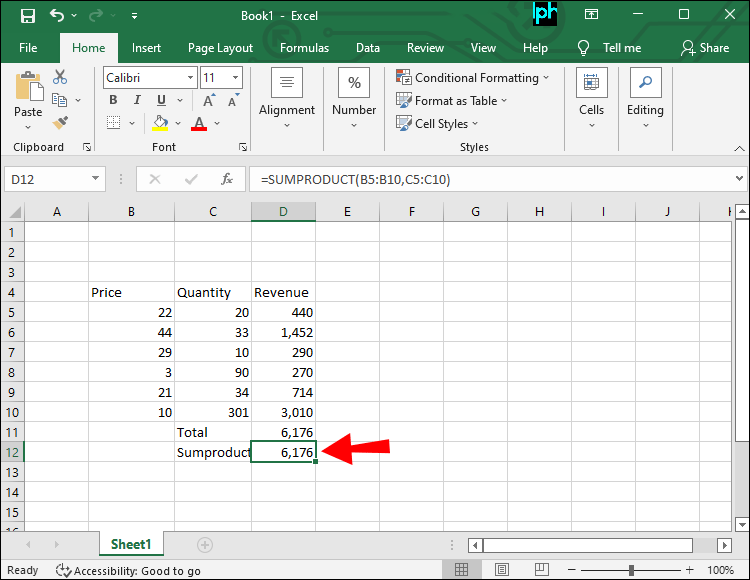5. The cell will now have a number showing you how many unique values there are in the document.

You can also use another formula for the job.

1. Open an Excel file.2. Click on a blank cell.3. Paste this formula into the cell: `=SUM(IF(FREQUENCY(IF(LEN(BX:BX)>0,MATCH(BX:BX,BX:BX,0),""), IF(LEN(BX:BX)>0,MATCH(BX:BX,BX:BX,0),""))>0,1))`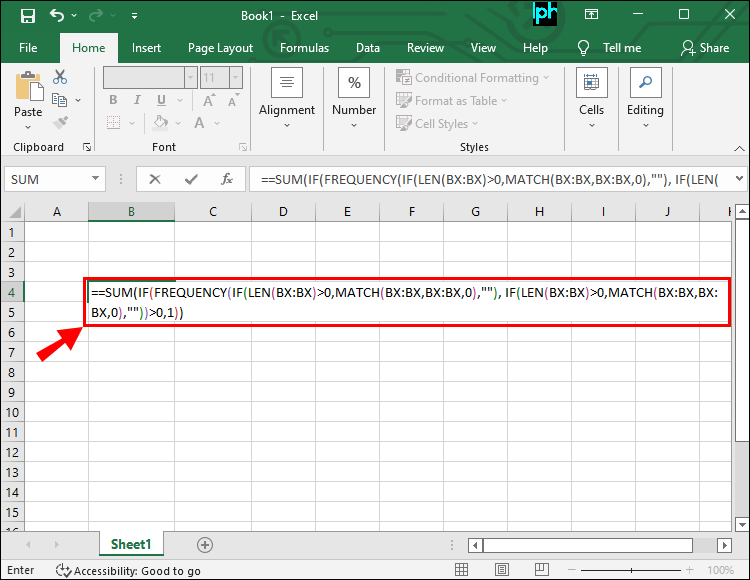4. Press the Control +Shift + Enter shortcut to run the formula.5. You’ll now see the number of unique values in the Excel file.

The numbers above are just a sample, so make sure you paste and type in which cells you want the formula to scan.

The first formula will only work with numbers. Therefore, if you’re dealing with unique values that include text, the second one will work.

If long formulas are too complicated, here’s a method using the “Advanced Filter.”2. Click on “Data.”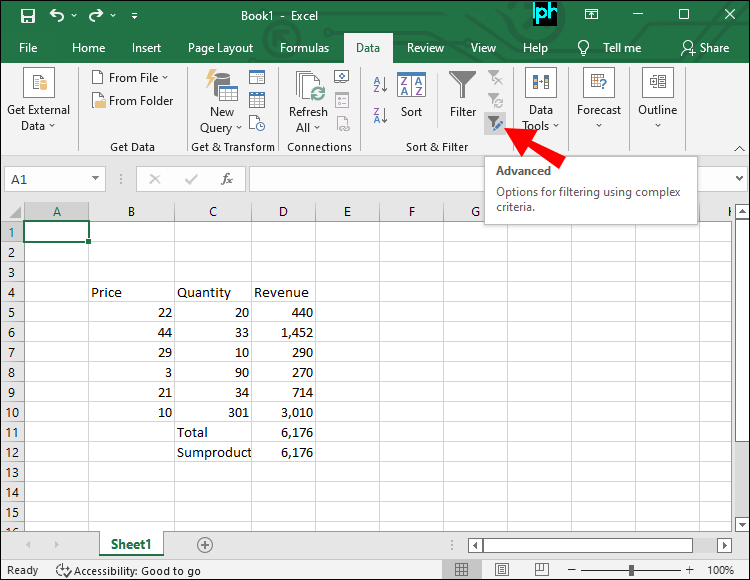4. Select “Copy To Another Location” in the box that appears” under “Action.”5. In the List Range, click on the icon on the right and select the range data to be filtered.6. For “Copy To,” pick a cell to place the filtered unique values.7. Check “Unique Records Only.”8. Select “OK” and click on another blank cell.9. Enter `=ROWS(XX:XX)`” and press the Enter key.10. The number of unique values will now be displayed to you.There are many different ways to check the number of unique values. You can also use Kutools. Follow the steps below to get started.

1. Install Kutools for Excel.2. Open Microsoft Excel.3. Select the range of data to analyze.4. Click on the Kutools icon and pick “Select.”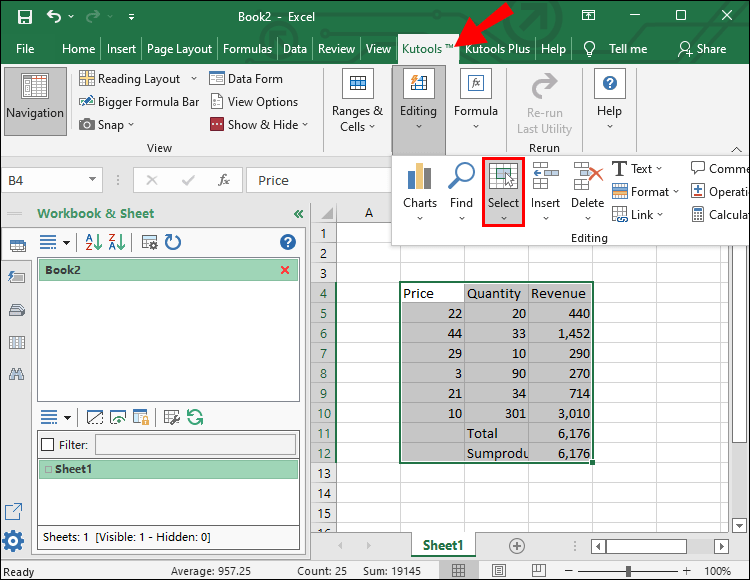5. Chose “Select Duplicate & Unique Cells.”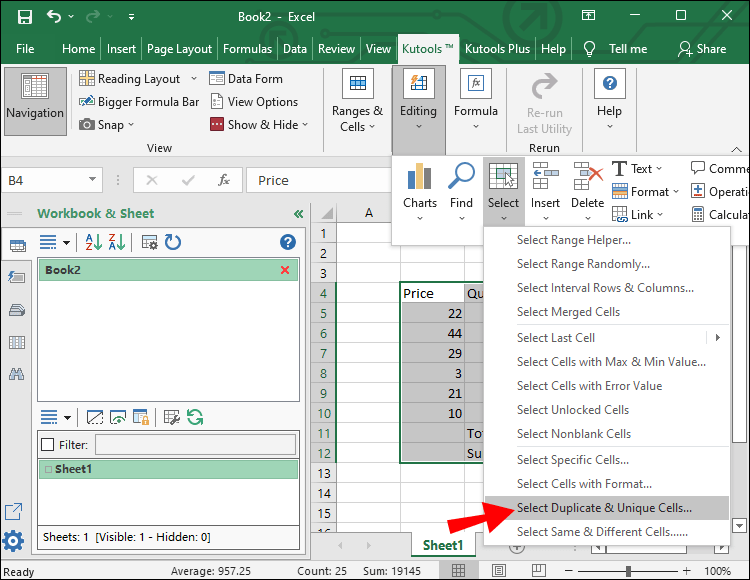Part 2

1. In this dialog box, click on “Unique Values Only.”2. Pick “Each Row.”3. Pick two colors under “Processing Of Results” to highlight the options.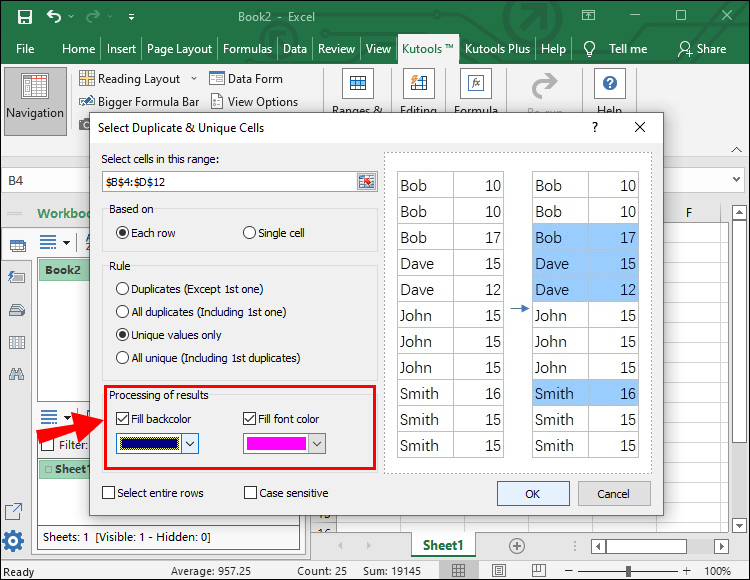4. Click on “OK.”6. You’ll now see all unique values highlighted.This method doesn’t show you how many there are, so you’ll need to count manually. However, it’s relatively simpler to use.

## How to Count Unique Values in Excel Using a Formula

We’ve been using some formulas in the previous sections, but there are many more you might prefer using.

### COUNTIF Function

The COUNTIF function works with numbers and words. Here are the instructions to use it:2. Determine the cells you want to be counted.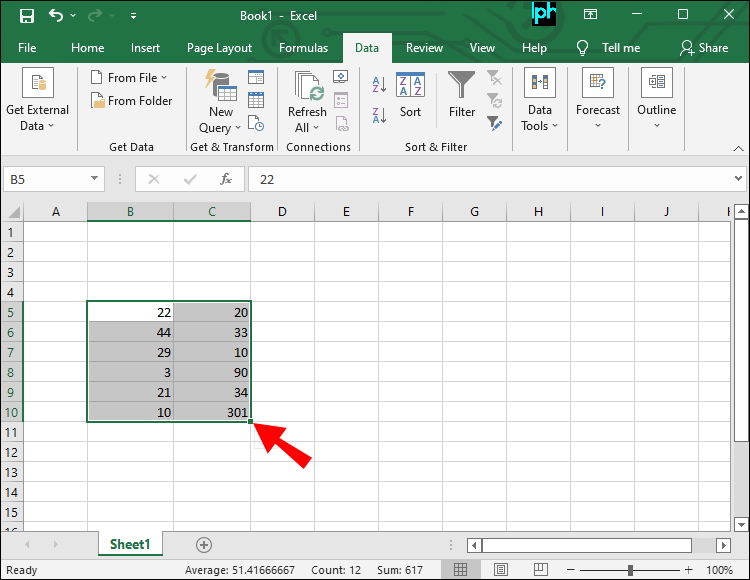3. In an empty one, enter the following: `=SUM(1/COUNTIF(XX:XX,XX:XX))`4. Finish the process by pressing the shortcut, Ctrl + Shift + Enter.As with the formulas above, XX stands for placeholder cells. You’ll need to enter the actual cell range manually.

There will be curly braces in the formula text box when you run it. Please don’t type these because they’re only present to indicate this is an array formula. When you edit it, they will vanish.

### SUMPRODUCT and COUNTIF

Another simple way to count these is to use a combination of the SUMPRODUCT and COUNTIF formulas.

1. Open Excel and pick a file.2. Check for the cells you want to count.3. Enter this formula in a blank cell: `=SUMPRODUCT(1/COUNTIF(XX:XX, XX:XX))`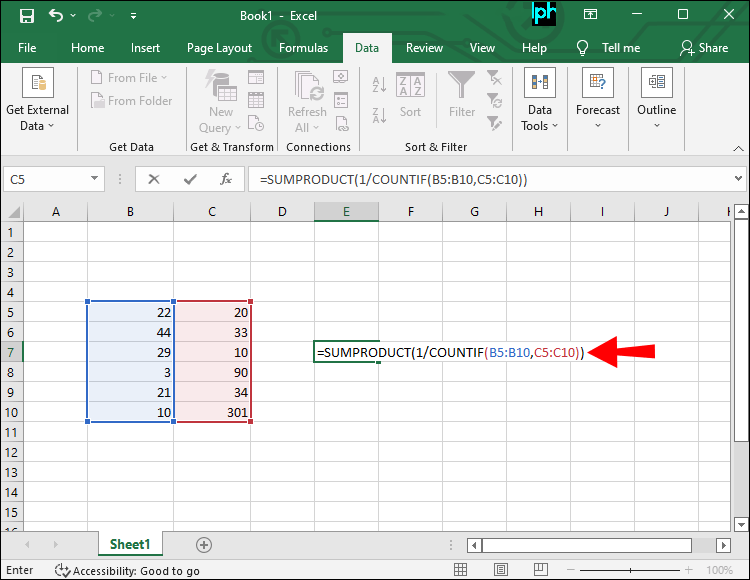4. Press Ctrl + Shift + Enter.Using this formula if there are blank cells in the data group is not recommended. You’ll get a “divide by zero” error if you do. The formula generates a zero for empty cells, and since dividing by zero is impossible, you’ll get an error.

A variant that ignores blank cells is as follows:

`=SUMPRODUCT(((XX:XX<>"")/COUNTIF(XX:XX , XX:XX &"")))`

This variant will ignore all blank cells, allowing you to find the unique values in the file.

### SUM, FREQUENCY, and MATCH

If your datasheet is extensive, the formulas above will become unresponsive. Instead of waiting, you can use this different formula.

`=SUM(IF(FREQUENCY(IF(XX:XX<>"", MATCH(XX:XX,XX:XX,0)),ROW(XX:XX)-ROW(firstcell)+1),1))`

“firstcell” will be the first cell containing data. This formula uses the FREQUENCY function and is an array formula. Therefore, curly braces will appear in Excel as you run the formula.

While the formula is more complicated, rest assured that it works very well.

## How to Count Unique Values in a Pivot Table

The Pivot Table brings a breath of fresh air to Microsoft Excel, as it’s a powerful tool for users to utilize. It can also let you get the unique values and distinct counts for a file.

Part 1

1. Click on any blank cell in an Excel file.2. Go to “Insert.”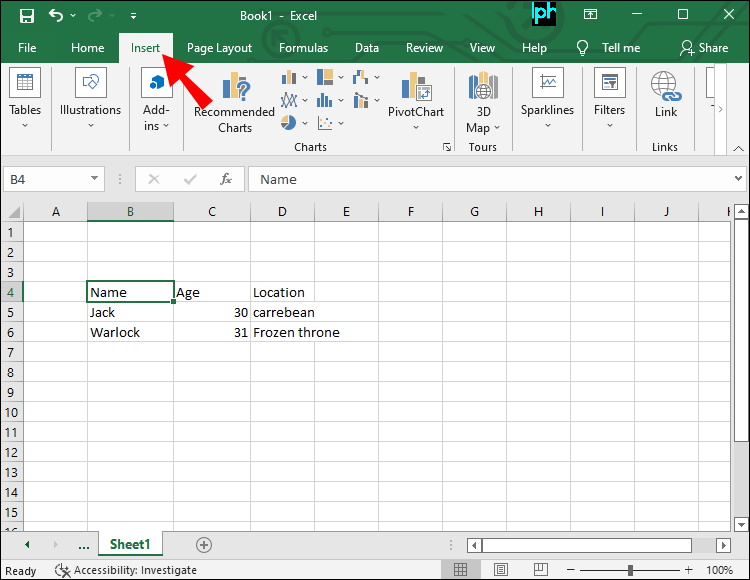3. Select “PivotTable.”4. In the dialog box, check “Add This Data To The Data Model.”5. At Table/Range, insert the range data you want.6. Click on “OK.”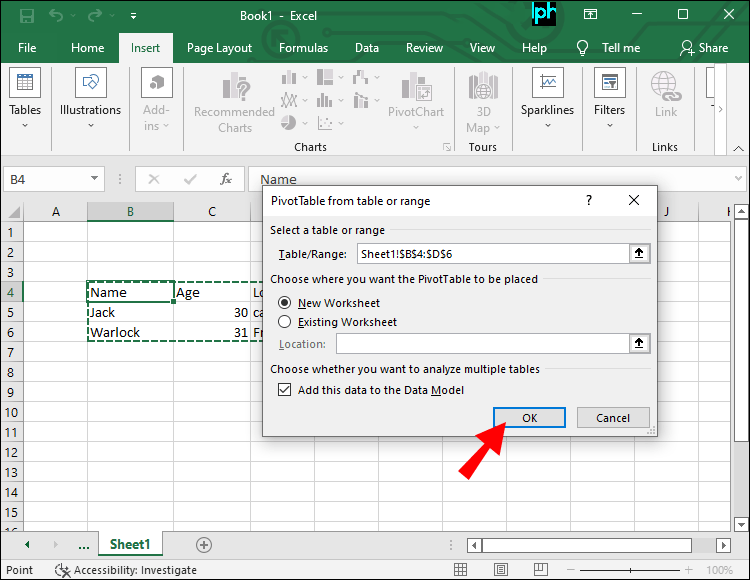Part 2

1. Click on the small arrow on the pivot table and drag it into the fields areas.2. Select “Value Field Settings.”3. Scroll down and find “Distinct Count.”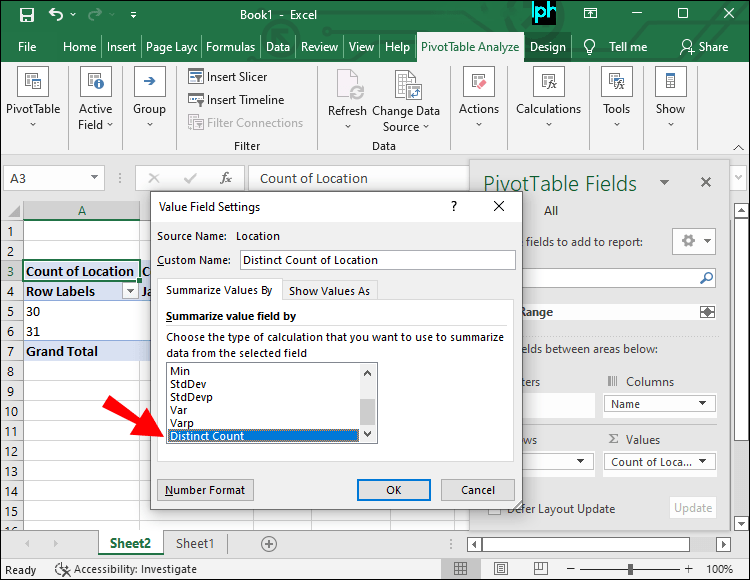4. Click on “OK” to confirm.5. Now you’ll have all the unique values and their distinct counts.

A pivot table isn’t as straightforward, but it does give you extra information like how many times a single value appears. It can help some people who need more than just unique values.

### Power Pivot

Power Pivot is one of the most powerful ways to get unique values. You may have to enable it if you’re using Excel 2010, but other versions don’t have this problem. Here’s how to get started:

Part 1

1. Click on the “Power Pivot” tab.2. Go to “Data Model” and click on “Manage.”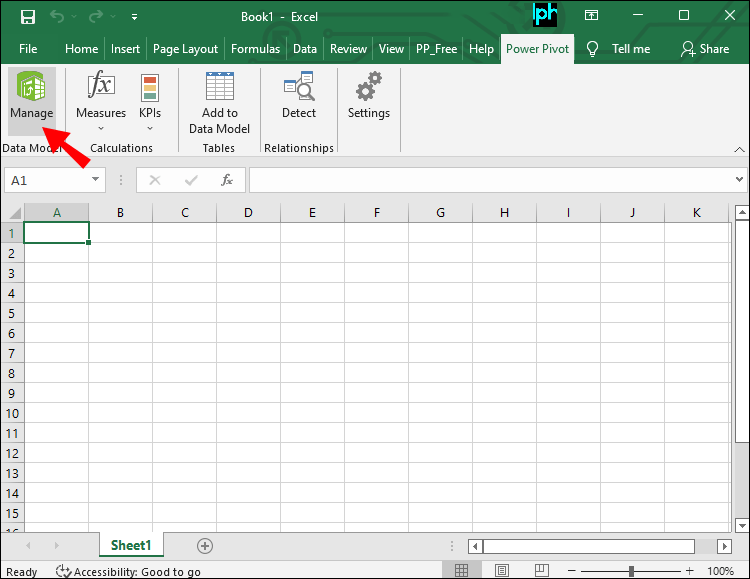3. A new window will open. Click on “Home.”4. Select “Get External Data.”5. Pick “From Other Sources.”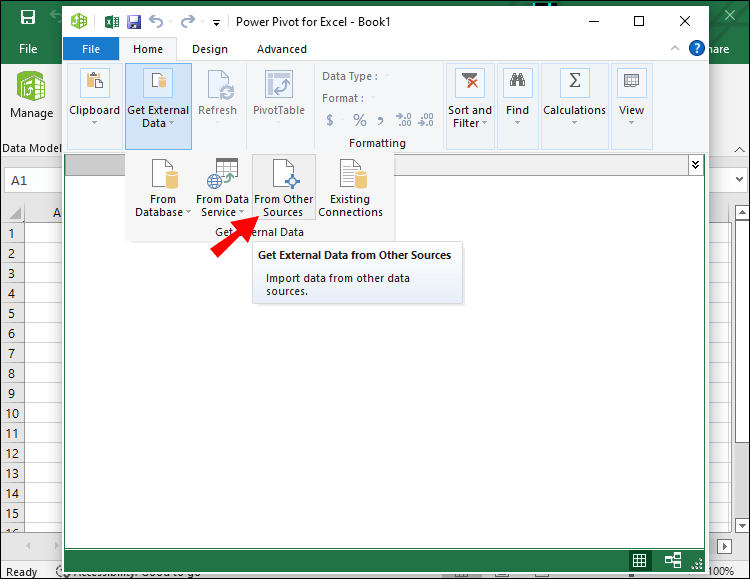6. Find the “Excel File” option.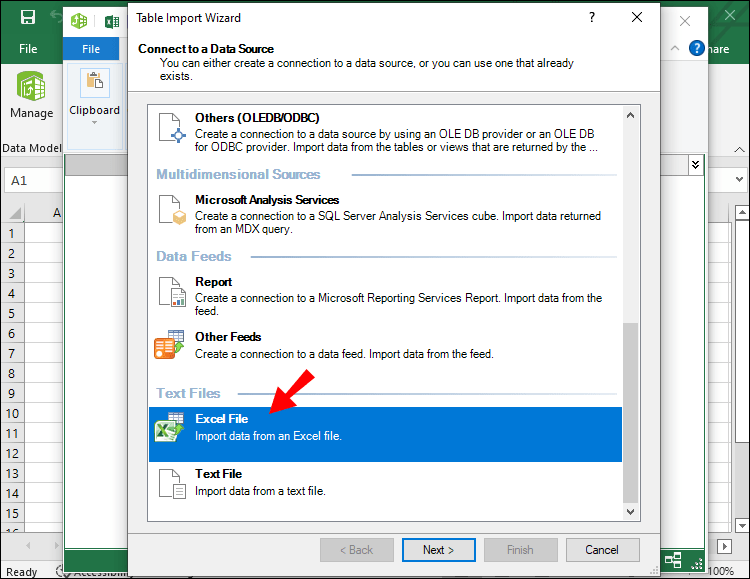7. Click on “Next.”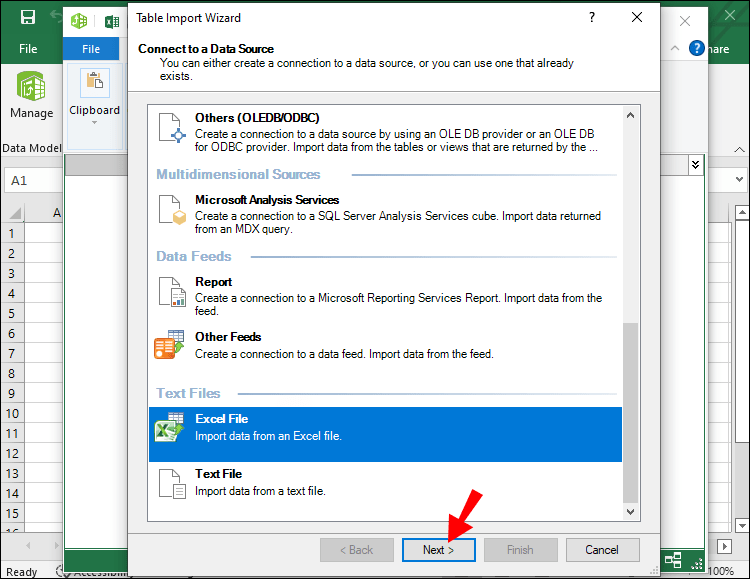8. Rename the connection (if you wish) and then select “Browse.”9. Find the Excel file you want to import.10. Click on “Use First Row As Column Headers” and go to the next section.Part 2

1. Finish importing the file to the data model and select “Finish.”2. Once the import operation concludes, close the import wizard.3. The information will now be in the Power Pivot.
4. Click on “PivotTable” and select “PivotTable.”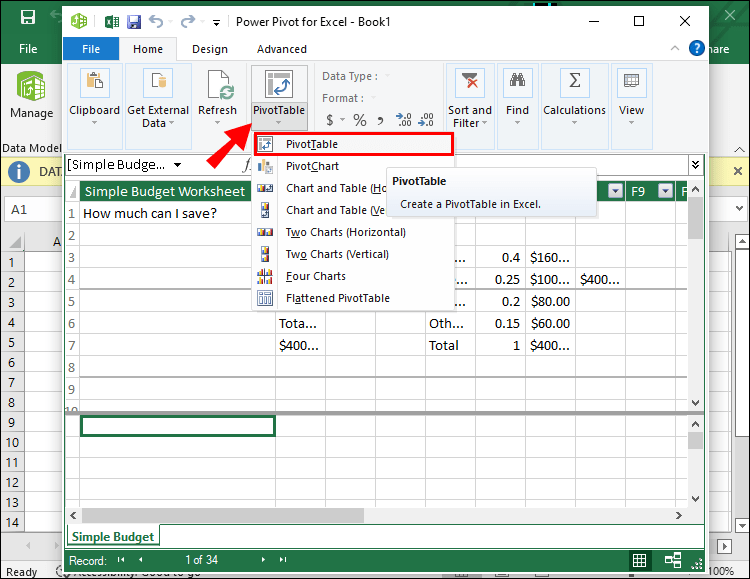5. Expand the datasheet in the popup.6. Place the unique values on the rows and distinct counts in another column.7. Create the pivot table.Part 3

1. Go to the Power Pivot tab and select “Measures.”2. Create a new measure.3. In “Formula,” type: `=DISTINCTCOUNT`4. Continue by adding a bracket to the end of the formula Select “UniqueValue[name]))5. The formula will now look similar to =DISTINCTCOUNT(Sheetname[Name]))6. Change the category to “Numbers.”7. Swap the “Format” to “Whole Number.”8. Choose “OK.”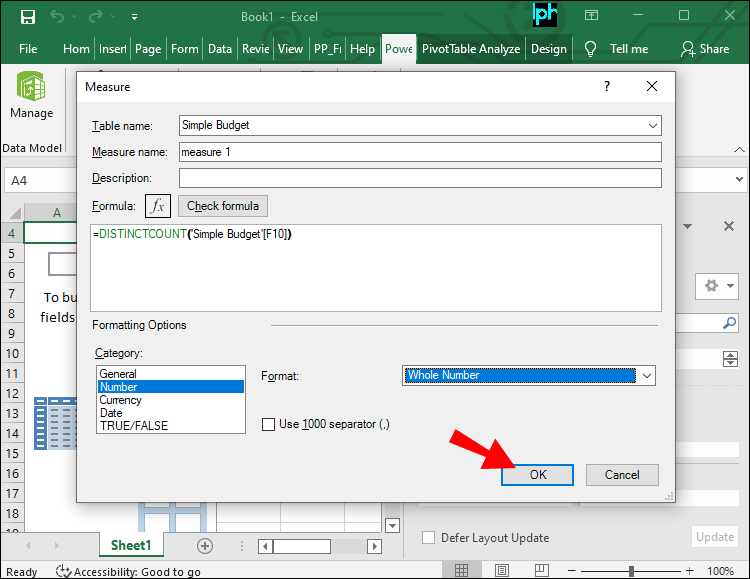9. Now, you can see all the unique values and distinct counts.## How to Count Unique Values Based On Multiple Criteria

If you have multiple criteria, it’s still possible to find how many unique values are there. You can use the COUNTIF formula for this job.

1. Look for the criteria you want to test for.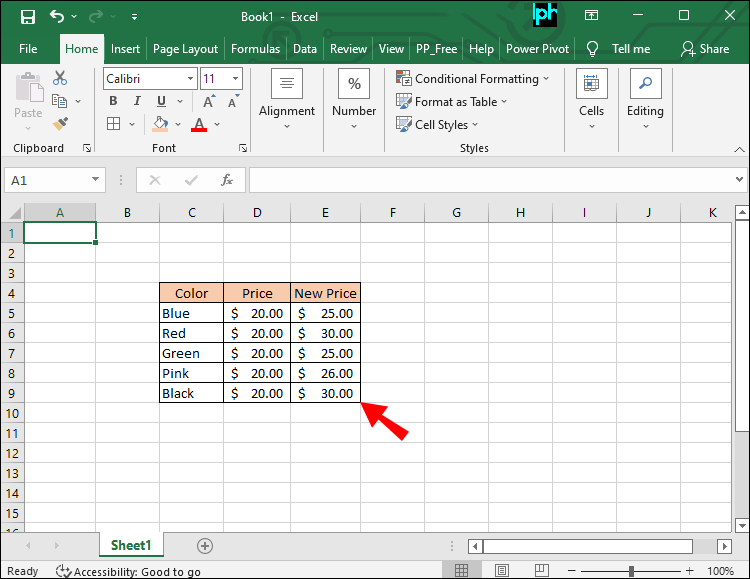2. Click on a blank cell.3. Type `=COUNTIFS(` and continue with the range you want to check.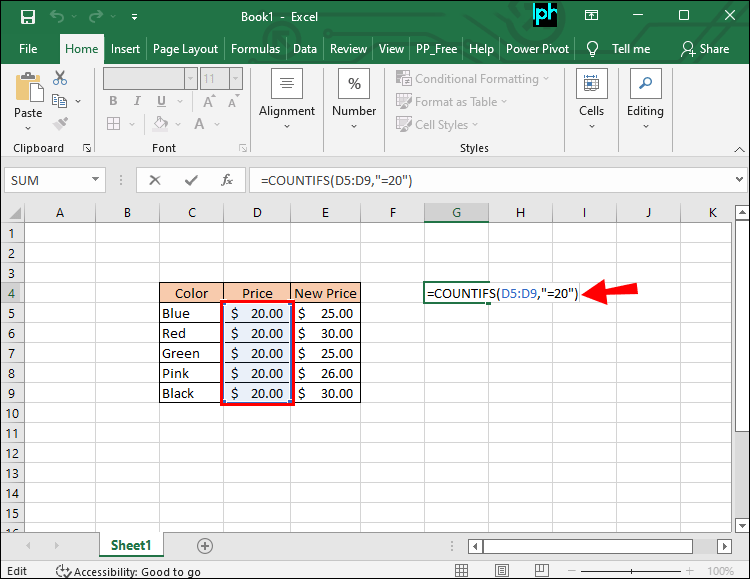4. Enter the test for the criteria.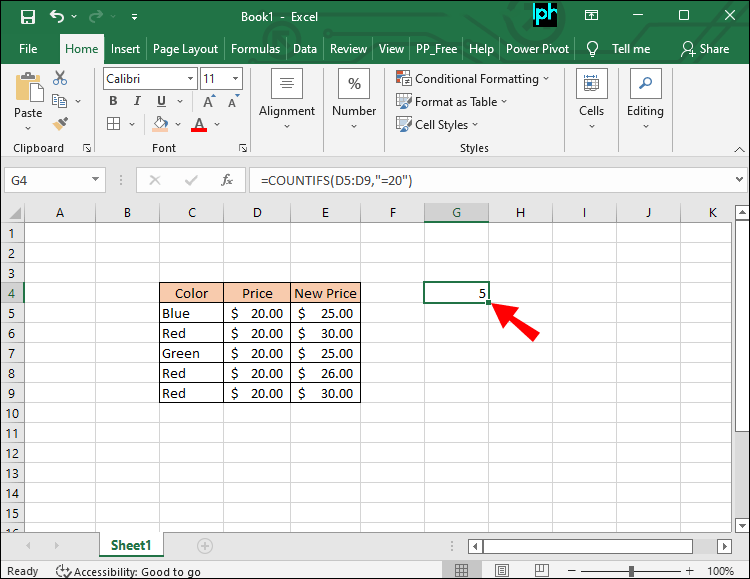5. Add a second range, which can be the same as before or a new one.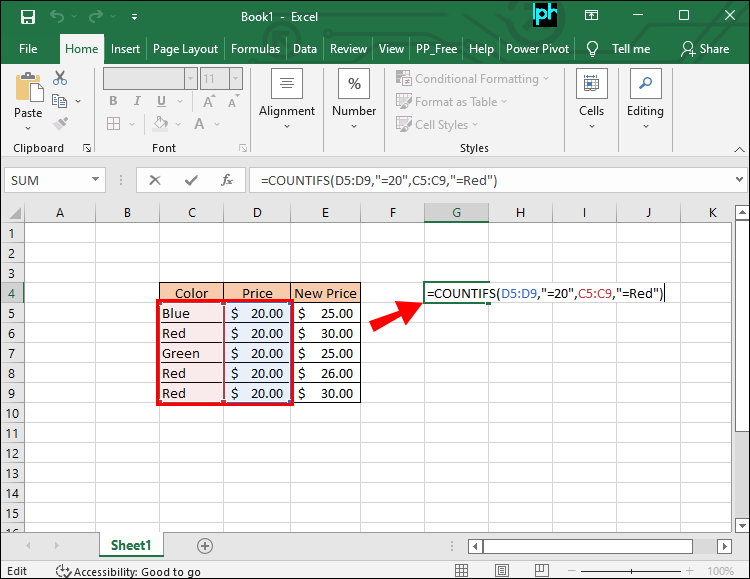6. Enter the test.7. Repeat as needed.
8. End with `)`and press the Enter key.

Your formula should look similar to this:

`=COUNTIFS(XX:XX,*>*&XX,XX:XX,*<&XX,XX:XX,*<&XX)`

This formula is handy and works with as many criteria as you like. Therefore, you can make it as long as the job requires.

## Interesting Numbers

Getting the unique values present in an Excel file shouldn’t be difficult and time-consuming. Experts have simplified this task to less than a minute with so many advanced features and available formulas. Of course, larger data sets will take time, but it’s still more convenient than counting manually.

Do you have a favorite method up there? What other ways do you know that aren’t listed here? Let us know in the comments section below.

Disclaimer: Some pages on this site may include an affiliate link. This does not effect our editorial in any way.

Todays Highlights